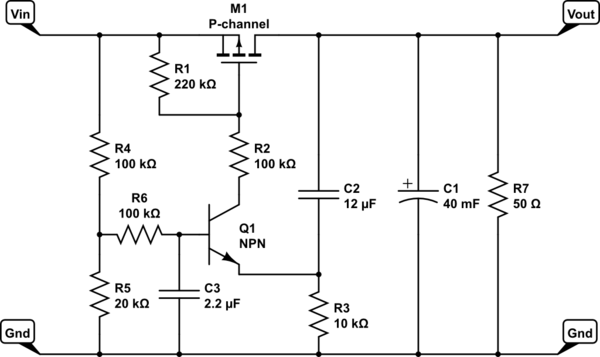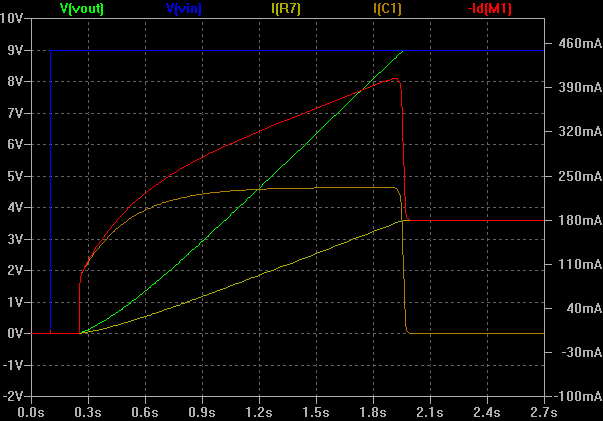# Issue 304: EQ AnswersProblem 1—The following circuit was designed to be an inrush current limiter for the large (40,000 µF) capaitor C1. R7 represents the application load of about 180 mA at 9 V.The load on the 9-V source (Vin) needed to be limited to about 350 mA, and the circuit performs remarkably well, as shown in the simulation. The current -Id(M1) is the drain current of the MOSFET.However, note that the circuit does not sense the source or load current directly. How then does it work?

Answer 1—Basically, the circuit works by using the C2-R3 combination as a model (or analog) for the charging of C1. Instead of sensing the current in C1 directly, R3 senses the current in C2, and it is assumed that this value is proportional to the current in C1, which is true as long as the voltage across R3 is a small fraction of the total.

Whenever there is a drop across R3 because of current through C2, the drive to the pass transistor is reduced.

The Thevenin equivalent of the base drive to Q1 is 1.5 V and 120 kΩ, so if the voltage across R3 ever rises as high as 1.5 V – 0.6 V = 0.9 V, Q1 is cut off altogether, removing the drive from M1 as well. Assuming a VBE for Q1 of 0.65 , this would occur at a C2 current of about 0.85 V/10 kΩ = 85 µA, which would correspond to a current in C1 of 85 µA × (40000 µF / 12 µF) = 280 mA.

By adjusting the resistor and capacitor values, you can change that limiting current value. Note that the total current through M1 (and the power supply) is the C1 charging currrent plus the rising load current through R7, so pick the limit value accordingly.

This analogy works because the basic equation of a capacitor says that the current through a capacitor is proportional to the rate of change of the voltage across it, and also to its capacitance:

i(t) = C dV(t) / dt

The assumption is that the voltage across R3 is “small”, which means that V(t) is essentially the same for both capacitors. This means that the current through each is directly proportional to its capacitance.

In this specific case, the voltage across R3 can be as high as 0.85 V, which is aobut 10% of the supply voltage, so the proportionality isn’t as precise as it could be, but it’s good enough for this purpose.

Problem 2—The actual charging current seems to be limited to about 230 mA. Why is this?

Answer 2—Transistor Q1 also contributes to the current flowing through R3, which means that the current through C2 must be corresponding less, which means that the current through C1 must be less, too.

Working backward, if the current through C1 is 230 mA, then the current through C2 would be about 230 mA × (12 µF / 40,000 µF) = 69 µA.

That implies that Q1 is passing about 85 µA – 69 µA = 16 µA.

This current passing through R1 would create a voltage drop of about 16 µA × 220 kohm = 3.52 V, which represents the threshold voltage of M1.

#### Problem 3—What is the function of C3?

Answer 3—C3 provides a “soft start” function for the circuit. If the base of Q1 were to rise instantly to 1.5 V, then M1 would be turned on fairly hard, creating an initial load spike on the power source.

Note that Q1 can turn on M1, charging its gate capacitance through R2. However, M1 can only be turned off by dicharging that capacitacne through R1, making turn-off much slower than turn-on.

As noted before, these resistances need to be high so that the current that they contribute to R3 is small relative to the current through C2.

Problem 4—Are there any special considerations on the selection of M1?

Answer 4—As long as M1 can handle the voltage and the current, and that the maximum VGS it sees is controllede by appropriate selection of R1 and R2, there’s really nothing special required.

Pay attention to the SOA (safe operating area) diagram in the datasheet. Plot some sample voltage and current values from the simulation in order to make sure it stays in the safe area.

Also, be sure to give it an adequate way to dissipate the pulse of heat associated with the charging surge of C1 without having its temperature rise too high.

 Keep up-to-date with our FREE Weekly Newsletter! Don't miss out on upcoming issues of Circuit Cellar. Subscribe to Circuit Cellar Magazine Note: We’ve made the May 2020 issue of Circuit Cellar available as a free sample issue. In it, you’ll find a rich variety of the kinds of articles and information that exemplify a typical issue of the current magazine. Would you like to write for Circuit Cellar? We are always accepting articles/posts from the technical community. Get in touch with us and let's discuss your ideas.